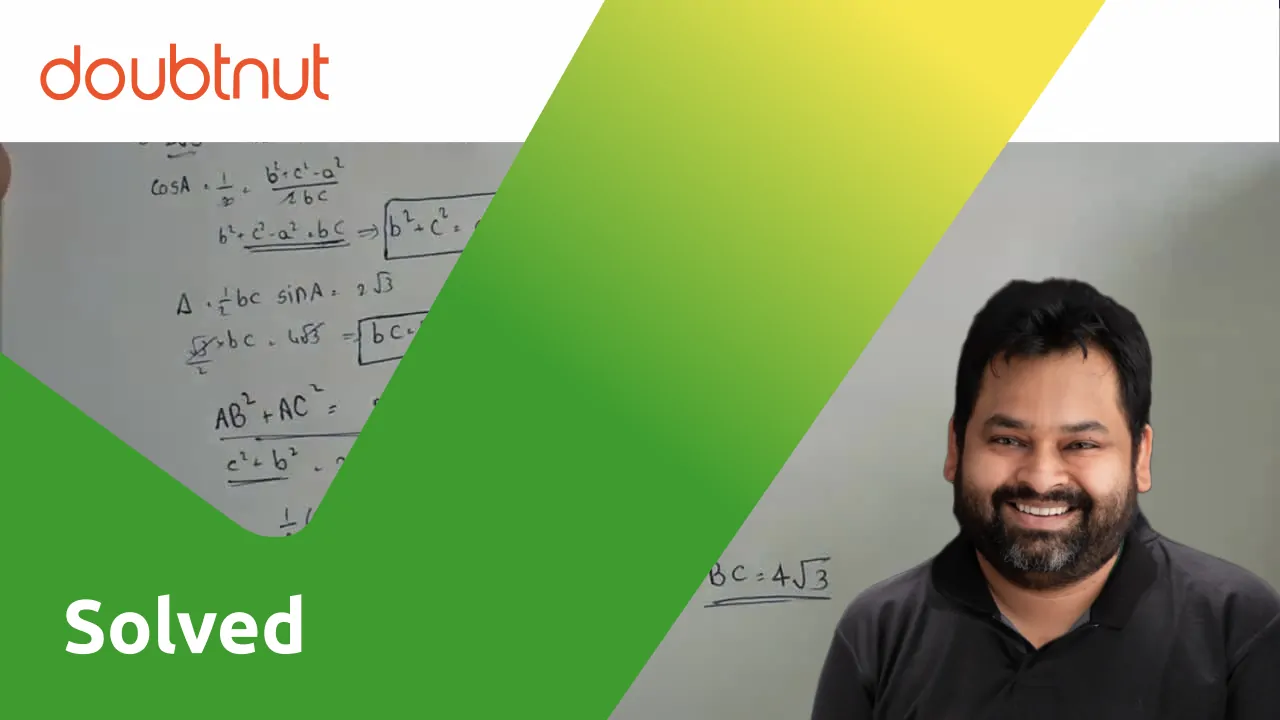Doubtnut App - Get 50 Lakh+ video solutions better than GoogleInstall Now

# In a !ABC the length of the median AD to the side BC is 4 units. If ∠A=60∘ and the area of the triangle is 2√3 sq. units. The length of side BC, is

A

23

B

43

C

6

D

8

Text Solution
Verified by Experts

## We have, ∠A=60∘ ⇒cosA=12⇒b2+c2−a22bc=12⇒b2+c2=bc…(i) It is given that Δ=2√3 sq. units ⇒12bcsinA=2√3⇒12bc×sin60∘=2√3 ⇒bc=8From (i) and (ii), we get b2+c2−a2=8⇒b2+c2=a2+8 In ΔABD, we have AB2+AC2=2(AD2+BD2) ⇒c2+b2=2(16+a24)⇒2(b2+c2)=64+a2 From (iii) and (iv), we get 2(a2+8)=64+aa2⇒a2=48⇒a=4√3